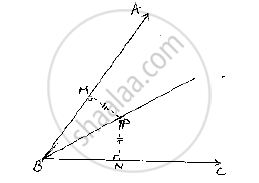Share
Notifications

View all notifications
Books Shortlist
Your shortlist is empty

# If Perpendiculars from Any Point Within an Angle on Its Arms Are Congruent, Prove that It Lies On the Bisector of that Angle. - CBSE Class 9 - Mathematics

Login
Create free account

Forgot password?
ConceptCriteria for Congruence of Triangles

#### Question

If perpendiculars from any point within an angle on its arms are congruent, prove that it lies on the bisector of that angle.

#### Solution

Given that, if perpendicular from any point within, an angle on its arms is congruent, prove that it lies on the bisector of that angle
Now,
Let us consider an angle ABC and let BP be one of the arm within the angle

Draw perpendicular PN and PM on the arms BC and BA such that they meet BC and BA in N and M respectively.

Now, in ΔBPM and ΔBPN
We have ∠BMP= BNP = 90°     [given]

BP=BP                                  [Common side]

And MP=NP                            [given]

So, by RHS congruence criterion, we have

ΔBPM≅ΔBPN

Now,

∠MBP=∠NBP        [ Corresponding parts of congruent                                            triangles are equal]

⇒ BP is the angular bisector of ∠ABC

∴ Hence provedIs there an error in this question or solution?

#### APPEARS IN

Solution If Perpendiculars from Any Point Within an Angle on Its Arms Are Congruent, Prove that It Lies On the Bisector of that Angle. Concept: Criteria for Congruence of Triangles.
S